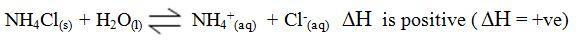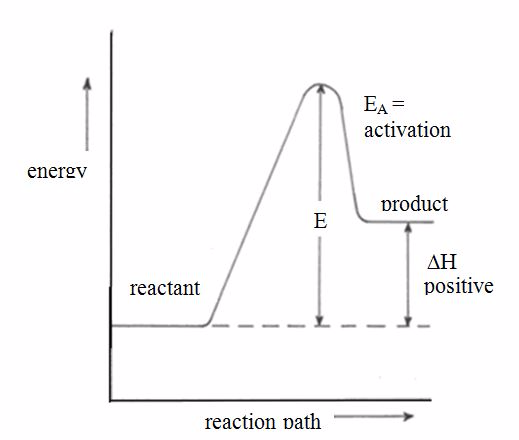error: Content is protected !!

# CHEMISTRY: FORM THREE: Topic 7 - CHEMICAL KINETICS, EQUILIBRIUM AND ENERGETICS

TOPIC 7: CHEMICAL KINETICS, EQUILIBRIUM AND ENERGETICS

Comparison between the Rates of Chemical Reactions
Compare the rates of chemical reactions
Chemical reactions take place at different rates. Some are fast whereas others are very slow. Let us consider the following reactions:
1. Addition of sodium metal to water: 2Na(s) + 2H2O(l) → 2NaOH(aq) + H2(g) The reaction takes place immediately and violently. It is therefore a fast reaction.
2. The rusting of iron in the presence of air and water giving hydrated iron (III) oxide, F2O3.XH2O: This is an extremely slow reaction.
These two reactions could be taken as representative examples of extremely fast and extremely slow reactions, respectively.There are, however, other reactions which proceed at rates intermediate between these two extremes. Rates of some of these reactions can be measured.
The rate of a chemical reaction can be measured in various ways. Let us consider the reaction between zinc and sulphuric acid to produce zinc sulphate and hydrogen gas:
Zn(s) + H2SO4(aq) → ZnSO4(aq) + H2(g)
When zinc is added to dilute sulphuric acid in a flask, they react together. The zinc slowly disappears and the gas (H2) bubbles off. After sometime, the bubbles of a gas form less quickly. The reaction is slowing down. Finally, no more bubbles appear. The reaction is over, because all the acid has been used up. Some zinc remains behind in a beaker.
In this reaction both zinc and sulphuric acid get used up in the reaction. At the same time, zinc sulphate and hydrogen form. The rate of this reaction could be determined by measuring any of the following:
• the amount of zinc used up per unit of time;
• the amount of sulphuric acid used up per unit of time;
• the amount of zinc sulphate produced per unit of time; or
• the amount of hydrogen produced per unit of time.
In general, the rate of a chemical reaction is determined by measuring the amount of reactant used up per unit of time or the amount of product produced per unit of time. Therefore, the rate of a chemical reaction simply refers to the amount of reaction which occurs in a unit time.

Experiments to Measure the Rates of Chemical Reactions
Perform experiments to measure the rates of chemical reactions
For the reaction described above, it is easiest to measure the amount of hydrogen produced per minute. The hydrogen can be collected as it bubbles off and its volume can then be measured as shown in figure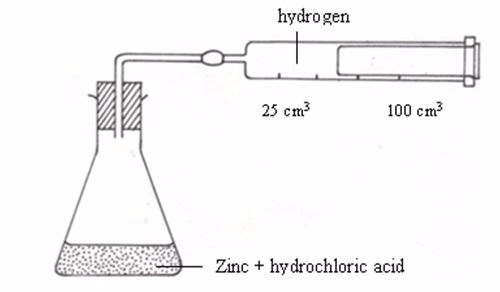Apparatus for measuring the production of gas
An experiment may be designed to measure the volume of hydrogen produced after every twenty seconds or so and then recording the data in a notebook.The table below shows sample results from such an experiment.
 Time (s) Volume of hydrogen gas (cm3) 0 0 20 13 40 22 60 30 80 37 100 41 120 44 140 46 160 47 180 47 200 47
Questions from the experiment
Use these data to draw a graph of time (horizontal axis) against volume of hydrogen (vertical axis).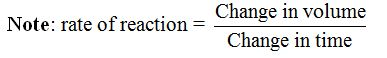There are also other ways by which rates of chemical reactions can be measured. These include measuring the:
1. change in intensity of colour: Many chemical reactions involve a change in colour. Potassium permanganate, for example, when it reacts with sulphur dioxide it changes from purple to colourless. The rate of such a reaction could be determined by measuring the rate at which the colour changes.
2. formation or disappearance of a precipitate: The reaction between hydrochloric acid and sodium thiosulphate produce a yellow precipitate of sulphur. The rate at which this precipitate forms is a measure of the rate of a reaction.
The Effect of Concentration on the Rate of a Reaction
Describe the effect of concentration on the rate of a reaction
A chemical reaction will occur only if the particles of the reacting substances are allowed to come in contact. Increasing the concentration means increasing the density of the particles and hence the probability of particles being close together and colliding more often. Thus, a reaction can be made to go faster or slower by changing the concentration of a reactant.
The effect of concentration on the rate of reaction can be determined by mixing dilute hydrochloric acid with sodium thiosulphate solution to produce a precipitate of sulphur.Since this reaction produces a precipitate from two different colourless solutions, the intensity of the precipitate at any given moment in time represents the extent of the reaction.
The experiment to determine this effect is carried out by mixing 2M hydrochloric solution acid with 50 cm3 of the thiosulphate solution (at different concentrations as shown in the table below) and noting the time taken by the cross to disappear using a stopclock. The procedure similar to that used to investigate the effect of temperature above is used except that, in this particular experiment, the concentration of the thiosulphate is altered each time the experiment is repeated. The following table shows the results.
 Concentration of thiosulphate (g/dm3) 10 20 30 40 50 Time(s) for the cross to disappear 250 120 65 32 15
As the results show, a reaction goes faster when the concentration of the thiosulphate (reactant) is increased. According to the above data, the rate of the reaction approximately doubles as the concentration of the thiosulphate is increased is increased by 10g dm-3.
The results clearly indicate that, as the concentration of the reaction is increased the time for the disappearance of the cross decreases, which chemically means that the rate of the reaction increases.
The Effect of Temperature on the Rate of a Reaction
Demonstrate the effect of temperature on the rate of a reaction
Increasing the temperature of the system means increasing the kinetic energy and hence the speed at which the reacting particles of the substance move. Thus, the particles collide more often and combine to form new substances. Temperature also provides the energy required to break the bonds of a substance and hence enhances the decomposition or splitting of a complex substance into more simpler substances. Therefore, an increase in the temperature of the system will result to an increase in the rate of reaction.
The effect of temperature on the rate of reaction can be determined experimentally. When dilute hydrochloric acid is mixed with sodium thiosulphate solution, a fine yellow precipitate of sulphur forms.
2HCl(aq) + Na2S2O3(aq) → 2NaCl(aq) + SO2(g) + S(s) + H2O(l)
The solid sulphur (S(s)) produced in this reaction makes the colourless solution go cloudy. The reaction is usually carried out in a flask placed on a piece of white paper, with a black cross marked on it. An experiment is designed such that each time a different temperature is used, while keeping the concentrations of the reactants constant.
At the beginning of the reaction, the cross can be seen easily. As the reaction goes on, more and more sulphur is deposited, the flask becomes more and more cloudy and the cross gradually gets harder to see. At last, the cross can no longer be seen. It is fully covered by the precipitate of sulphur. Time taken by the cross to disappear at a given temperature indicates the rate of reaction at that temperature. The quicker the disappearance of the cross, the faster is the reaction and vice versa.
The reaction rate can be determined by the following procedure:
1. 50 cm3 of a solution of sodium thiosulphate (containing 40g per litre of the solution) is measured and put into a 100 cm3 beaker.
2. A wire gauze is placed on a tripod stand, then the beaker is put on the gauze, and the solution is gently warmed with a Bunsen burner flame.
3. A thick black cross is marked on a white piece of paper or cardboard.
4. When the temperature reaches a little above 200C, the beaker is taken quickly and placed on the cross.
5. 10 cm3 of 2M hydrochloric acid solution is added quickly to the beaker and a clock is started at the same time. The temperature of the mixture is noted.
6. When the cross is no longer visible, the clock is stopped.
7. The experiment is repeated more times at temperatures of 300C, 400C, 500C, 600C, etc. Each time 10 cm3 of 2M hydrochloric acid and 50 cm3 of the thiosulphate solution are used.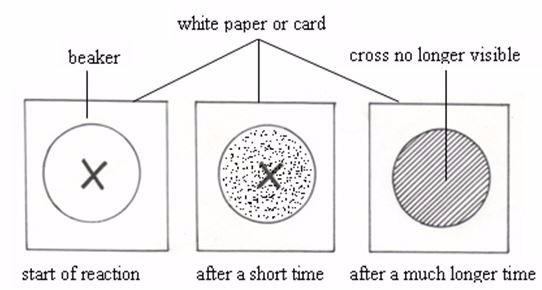Measuring the effect of temperature on the rate of reaction
The following table shows the specimen results obtained from such an experiment:
 Temperature (0C) 20 30 40 50 60 Time (s) for cross to disappear 200 125 50 33 24
The results show that the higher the temperature the faster the cross disappears, which in turn, means that the rate of the reaction increases.Therefore, the data clearly indicate that a reaction goes faster when the temperature is raised. When the temperature is increased by 100C, the rate approximately doubles.

The Effect of Surface Area of a Solid on the Rate of a Reaction
Show the effect of surface area of a solid on the rate of a reaction
In many reactions, one of the reactants is a solid. The reaction between hydrochloric acid and calcium carbonate (marble chips) is one example. Carbon dioxide gas is produced.
CaCO3(s) + 2HCl(aq) → CaCl2(aq) + H2O(l) + CO2(g)
One of the ways by which the rate of such a reaction can be increased is by reducing the particle size of the solid substance (marble chips). If this substance is grinded to fine powder or to small pellets, the surface area of the marble is increased. Therefore, more of the marble is exposed to the acid for efficient reaction. This leads to increase in the rate of reaction. Increased rate of reaction results to increased production of carbon dioxide gas. Hence, the rate of reaction can be determined by measuring the time taken to produce a given volume of carbon dioxide by reacting equal masses of whole marble and crushed (or powdered) marble, and then comparing the two results. The data obtained is recorded in a table as shown below:
 Time (s) 0 10 20 30 40 50 60 70 80 90 100 Volume (cm3) Whole marble 0 18 24 32 38 45 53 62 72 80 80 Grinded marble 0 34 52 67 74 76 80 80 80 80 80
The data above shows that it takes 90 seconds for whole marble chips to react to completion while for powdered marble it takes only 60 seconds. Also there is seen a small increase in volume of carbon dioxide gas per unit of time in the case of ungrounded (whole) marble as compared to the powdered sample. This absolutely proves that an increase in the surface area of marble (a solid reactant) increases the rate of reaction and hence the rate of production of carbon dioxide gas.

The Effect of Catalyst on the Rate of a Reaction
Demonstrate the effect of catalyst on the rate of a reaction
A catalyst usually increases the rate of a reaction and this is called positive catalysis. In general, a catalyst will function even if present in very small amounts. Hydrogen peroxide is a clear, colourless liquid. It can decompose to water and oxygen:
2H2O2(aq) → 2H2O(l) + O2(g)
The rate of decomposition (reaction) of the peroxide can be increased tremendously by adding a very little of manganese (IV) oxide in the reaction vessel.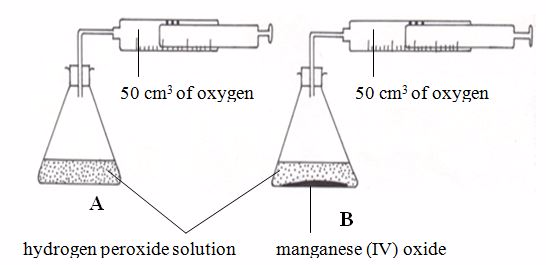Investigating the effect of catalyst on rate of reaction
The reaction in flask A above is in fact very slow. It could take several days to produce just 50 cm3 of oxygen. In flask B only 1g of manganese (IV) oxide is added. The reaction goes very faster. 50 cm3 of oxygen is produced in a few minutes.
The manganese (IV) oxide speeds up the reaction without being used up itself. It is called a catalyst for the reaction.A catalyst is a substance that changes the rate of a chemical reaction but remains chemically unchanged at the end of the reaction.
Catalysts for many reactions have been discovered. They are usually transition metals or compounds of transition metals. There are also biological catalysts, called enzymes. For example, the pancreatic juice secreted by the pancreas contains enzymes that speed up digestion process.
Examples of catalysts for some common reactions are given in the following table:
 Reaction Catalyst Heating of potassium chlorate 2KClO3(s) → 2KCl(s) + 3O2(g) Manganese (IV) oxide (MnO2) Synthesis of sulphur trioxide 2SO2(g) + O2(g)⇔2SO3(g) Vanadium (IV) oxide (V2O5) 1. Synthesis of ammonia Reduced iron powder 2. Decomposition of hydrogen peroxide Manganese (IV) oxide or platinum powder

Comparison between Reversible and Irreversible Reactions
Compare reversible and irreversible reactions
Chemical reactions can be classified into two groups, that is, reversible and irreversible reactions.
Irreversible reactions are those reactions which go to completion. In such reactions, a known product is formed. The product(s) cannot be reversed back to the original reactant(s). Consider the reaction between sodium and chlorine to produce sodium chloride: 2Na(s) + Cl2(g) → 2NaCl(s)
You cannot turn sodium chloride back to chlorine gas and pure sodium metal by ordinary means. This is a typical irreversible reaction.
Several reactions are known to proceed in both directions, provided certain conditions are maintained.A + B⇌AB Such reactions are said to be reversible. The sign ⇌indicates reversibility.
The Concept of Reversible and Irreversible Reactions
Describe the concept of reversible and irreversible reactions
A reversible reaction is a chemical reaction in which the products can react to re-form the reactants.In such reactions, reactants combine to form products. However, under certain conditions, the products may be converted back to reactants.The idea of “reactants” and “products” in such circumstances is really confusing.
Example 1
Examples of reversible reactions
1. When you heat blue crystals of copper (II) sulphate, they breakdown into anhydrous copper (II) sulphate, a white powder: CuSO4.5H2O(s)⇌CuSO4(s) + 5H2O(g)The reaction can be reversed by just adding water to the white powder, which quickly turns to blue crystals again. In fact, this is used as a test for water: CuSO4(s) + 5H2O(l)⇌CuSO4.5H2O(s)
2. When you heat ammonium chloride (a solid) in the bottom of a test tube, it breaks down into ammonia and hydrogen chloride (gases). The gases readily combine at the top of the tube where it is cool: NH4Cl(s)⇌NH3(g) + HCl(g)This is a reversible reaction.

Difference between Equilibrium Reactions and Simple Reversible Reactions
Differentiate equilibrium reactions from simple reversible reactions
Suppose that 2 molecules of substance A reacts with 3 molecules of substance B to produce 1 and 2 molecules of substances C and D respectively in a homogenous system (i.e. entirely liquid or entirely gaseous).
2A + 3B⇌C + 2D
As soon as a little of C and D are formed, a reverse reaction will begin. At first the forward reaction will predominate, but, as C and D accumulate the reverse reaction will build up until an equilibrium position is reached, with forward and reverse reactions proceeding at the same rate. The composition of the mixture will then appear constant, though it is the net result of the two opposing reactions. Since chemical equilibrium involves the balancing of two reactions which are proceeding at the same time in opposite directions, it is said to be a dynamic equilibrium, that is, it is an equilibrium involving the constant interchange of particles in motion. Equilibrium is a dynamic condition in which two opposing changes can occur at equal rates in a closed system. In a closed system, matter cannot enter or leave, but energy can. Both matter and energy can escape or enter an open system. For example, sunlight, heat from a burner, or cooling by ice can cause energy to enter or leave a system.
An example of a real equilibrium reaction is the decomposition of mercury (II) oxide. Mercury (II) oxide decomposes when heated to produce mercury and oxygen gas.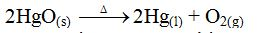Again, mercury and oxygen combine to form mercury (II) oxide when heated gently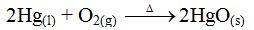Suppose mercury (II) oxide is heated in a closed system. Once the decomposition has begun, the mercury and oxygen released can re-combine to form mercury (II) oxide again. Thus, both reactions can proceed at the same time. Under these conditions, the rate of the composition (re-combination) reaction will eventually equal that of the decomposition reaction. Then the reaction is said to be at equilibrium. At equilibrium, mercury and oxygen will combine to form mercury (II) oxide at the same rate that mercury (II) oxide decomposes into mercury and oxygen. The amounts of mercury (II) oxide, mercury and oxygen can then be expected to remain constant as long as these conditions persist. At this point, a state of dynamic equilibrium has been reached between the two chemical reactions. Both reactions continue, but there is no net change in the composition of the system.
A reversible reaction is in chemical equilibrium when the rate of its forward reaction equals the rate of its reverse reaction, and the concentrations of its reactants and products remain unchanged.
Two Equilibrium Reactions of Industrial Importance
Describe two equilibrium reactions of industrial importance
Conditions for the industrial synthesis of different substances have to be carefully chosen if the process is to be efficient and thus economically viable. The considerations are:
• Yield – how much yield is produced?
• Rate – how fast is it produced?
• Energy – how much energy is lost during the process?
The way in which this is achieved in practice is described briefly for three important industrial reactions, namely, the Haber Process for the manufacture of ammonia, the Contact Process for the manufacture of sulphuric acid and the thermal dissociation of calcium carbonate for the industrial manufacture of lime.
THE HABER PROCESS FOR THE INDUSTRIAL MANUFACTURE OF AMMONIA
The Haber process involves the reaction between nitrogen and hydrogen to produce ammonia:
N2(g) + 3H2(g)⇌2NH3(g)
A German Chemist, Fritz Haber, was the first to show how this reaction could be controlled to make useful amounts of ammonia. The first industrial plant making ammonia by the Haber process opened in Germany in 1913. Now over 100 million tones of ammonia are produced each year by this process. Because of its importance, the Haber process for making ammonia has been studied over a wide range of conditions of temperature and pressure.
The effect of pressure
Ammonia is produced from its elements by reduction of volume. Therefore, if the system is in equilibrium and the pressure is raised, the equilibrium will shift to the right. This is because the system will shift to favour the side of the equation that has fewer molecules.
N2(g) + 3H2(g)⇌2NH3(g)
Therefore, high pressure will increase the yield of ammonia. Modern industrial plants use a pressure of 200-500 atmospheres. Pressure higher than this range could be used, but high-pressure reaction vessels are expensive to build.
The effect of temperature
The forward reaction producing ammonia is exothermic and the reverse reaction is therefore endothermic. Therefore, lowering the temperature will favour ammonia production. However, the rate at which the ammonia is produced will be so slow as to be uneconomical. So, it is necessary to include a catalyst which will give sufficient reaction rate in spite of a relatively low pressure. In practice, a compromise or optimum temperature is used to produce enough ammonia at an acceptable rate. Modern plants use temperatures of about 4500C.
The effect of concentration
If the system is in equilibrium and more nitrogen is then added to increase its concentration in the reaction mixture, the equilibrium shifts to the right so as to tend to reduce the concentration of nitrogen. That is, more ammonia will be produced to use up nitrogen. Also, if hydrogen is added the equilibrium will, similarly, shift to the right.
However, in practice, there is no particular advantage in using excess of either material (nitrogen or hydrogen) since the gases, nitrogen and hydrogen, are mixed in a ratio of 3:1 by volume.Hydrogen is manufactured from partial combustion of hydrocarbons, and nitrogen is obtained from the air. They are mixed in the ratio of 3:1 proportion by volume and dried (e.g. by silica gel). They are pre-heated by gases leaving the catalyst chamber over the catalyst at 4500C. The ammonia produced is absorbed in water or liquefied by refrigeration and the remaining nitrogen and hydrogen gases are recycled.
If the system was at equilibrium and then some of the ammonia was removed, more ammonia would be produced to replace that which is removed. The gas is removed from the reaction chamber when the percentage of ammonia in the equilibrium mixture is 15%.
Based on the above discussions, the overall conditions used for the maximum production of ammonia are as follows:
• N2 and H2 are mixed in the ration of 3:1
• An optimum temperature of 4500C is chosen.
• A very high pressure (200-500 atm) is applied.
• A catalyst of finely divided reduced iron, usually promoted by alumina (aluminium oxide) is used.
• The ammonia is condensed and removed out of the reaction mixture and the remaining N2and Hrecycled.
THE CONTACT PROCESS FOR THE INDUSTRIAL MANUFACTURE OF SULPHURIC ACID
The first step in the production of sulphuric acid is the conversion of sulphur dioxide to sulphur trioxide. The process involves the reaction between sulphur dioxide and oxygen. The reaction is exothermic and reversible.2SO2(g) + O2(g)⇌2SO3(g)
To make sulphuric acid, the sulphur trioxide gas produced is dissolved in 98% concentrated sulphuric acid, forming “oleum” which is then diluted with the correct amount of water to give ordinary concentrated sulphuric acid.
• The effect of pressure: There are fewer gas molecules on the right of the equation. Therefore, increasing the pressure would favour the production of sulphur trioxide. In fact, the process is run at atmospheric pressure because the conversion of sulphur dioxide to sulphur trioxide is about 96% complete under these conditions. In practice, it is found that use of high pressure produces a very small gain in yield and involves extra cost. It is for this reason that ordinary atmospheric pressure is used.
• The effect of temperature: The reaction to produce sulphur trioxide is exothermic so, if the temperature is lowered the equilibrium shifts to the right. Hence, more sulphur trioxide will be produced. This means that sulphur trioxide production would be favoured by low temperatures. However, too low temperatures reduce the rate of reaction so increasing the time required for the production of the sulphur trioxide. This would mean an increase in production cost. For this reason, a catalyst must be introduced. A catalyst of vanadium (V) oxide is used to increase the rate of reaction. An optimum temperature of about 4500C is used. This gives sufficient sulphur trioxide at an economic rate. In general, low temperatures give an equilibrium favourable to an exothermic reaction, but catalysis is needed to give a favourable reaction rate.
• Effect of concentration of reactants: Suppose that in the contact process reaction, an equilibrium has been reached in certain conditions and oxygen is added to the system. According to Le Chatelier’s principle, the reaction would shift to the right so as to oppose this change, that is, to reduce the concentration of the oxygen added towards its former level. This can only be achieved by combining it with sulphur dioxide to form sulphur trioxide. So, increased concentration of oxygen favours conversion of more sulphur dioxide to trioxide. Likewise, a similar case can occur when sulphur dioxide is added to the system. In a similar manner, increased concentration of sulphur trioxide would favour the conversion of oxygen to sulphur trioxide and hence increased formation of the trioxide.In general, the conditions used in the contact process are as follows: An optimum temperature of about 4500C is chosen; A catalyst, vanadium (V) oxide, is used to speed up the reaction; An operating pressure of 1 atmosphere is applied. A higher pressure, which would theoretically increase the yield of SO3, is not used as it is uneconomical; Yield of SOwould be increased by increasing either SO2 or O2. The cheaper reactant is O2.

The Concept of Endothermic and Exothermic Reactions
Explain the concept of endothermic and exothermic reactions
You have met many different chemical reactions so far in chemistry. But they all have one thing in common, that is, they involve an energy change. The great majority of chemical reactions are accompanied by a marked heat change.
During chemical reactions as reactants form products, there is a change in heat content. This is referred to as the enthalpy change and is always expressed in kilojoules per mole (kJmol-1). Two types of heat change are distinguished. Those reactions that are accompanied by evolution of heat to the surroundings are termed as exothermic reactions while those that are accompanied by absorption of heat from the surroundings are endothermic reactions.
• An exothermic reaction is one during which heat is liberated to the surroundings.
• An endothermic reaction is one during which heat is absorbed from the surroundings
When magnesium is burnt in air heat is evolved.
2Mg(s) + O2(g) → 2MgO(s)+ heat
The same case applies to the burning of coal in air.
C(s)+ O2(g) → CO2(g) + heat
Mixing sulphur nitrate and sodium chloride solutions gives a white precipitate of silver chloride and a temperature rise.
AgNO3(aq) + NaCl(aq) → AgCl(s) + NaNO3(aq)
When ammonium nitrate is dissolved in water, there is a fall in temperature. Also adding a mixture of citric acid and sodium bicarbonate to water produces bubbles and a fall in temperature. In both reactions, the temperature of the water falls because the reactions take heat energy from it. These reactions are therefore endothermic.
The heat changes that occur during any chemical reaction represent changes in the energy content of the whole system. The energy content may increase or decrease depending upon whether heat is absorbed or evolved.
Energy Level Diagrams for Exothermic and Endothermic Reactions
Draw energy level diagrams for exothermic and endothermic reactions
For exothermic reactions, the enthalpy changeis conventionally assigned a negative value. For example, when pellets of sodium hydroxide or concentrated sulphuric acid dissolve in water, heat is evolved and the system loses heat to the surrounding.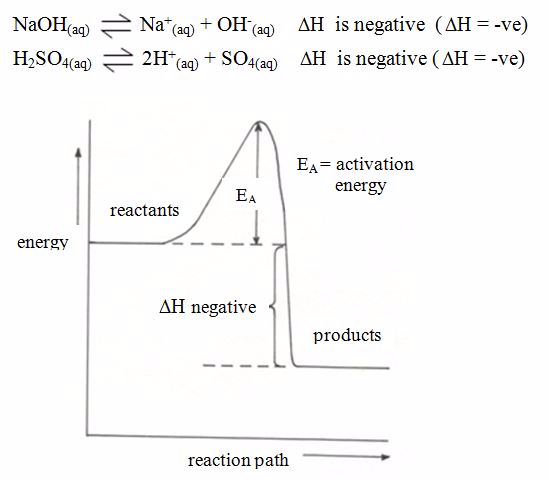Energy level diagram for exothermic reaction
For endothermic reactions, the enthalpy changeis assigned a positive value. For example, when potassium iodide or ammonium chloride dissolves in water, heat is absorbed from the surroundings.